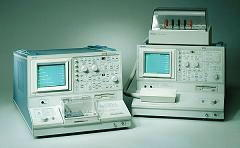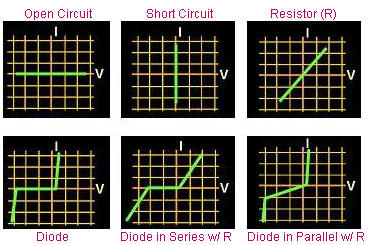Curve Tracing

Curve tracing is the process of analyzing the current-voltage characteristics of an electrical path using an equipment known as a curve tracer (see Fig. 1).  A standard curve tracer has a CRT screen that can show the behavior of the current as the voltage is varied.  The CRT display of a curve tracer consists of a grid that displays the current level along the y- axis and the voltage level along the x-axis. The intersection of the main (center) axes is the point where both the current through and the voltage across the selected electrical path are zero.

Curve tracing is very useful in failure verifications and in the early stages of failure analysis. It can identify electrical failures that exhibit abnormal voltage-current relationships at the output pins.   A curve tracer is usually used with two probes, one for each of the nodes that define the electrical path being characterized.Fig. 1.  Photo of Tektronix Curve Tracers

When performing curve tracing on an integrated circuit, one of the probes is usually attached to a reference pin as the other probe is rotated among the other pins, i.e., it is connected to each of the other pins one at a time.  The voltage-current (V-I) curve of each pin of the device under test (DUT) with respect to the reference pin is then compared to that exhibited by a known good device (KGD).  The analog ground (AGND), +Vs, and -Vs pins are usually chosen as reference pins since they are nodes that are, more often than not, common to all the other pins.

Interpreting a V-I curve is not difficult.  For example, an electrical path that projects a horizontal line at I=0 on the CRT display is an open circuit, since the current level remains at zero even if the voltage is varied from a negative to a positive value. On the other hand, a vertical line along V=0 indicates a short, since the voltage stays at zero regardless of the current level.  A purely resistive path would show a straight, diagonal line, with the reciprocal of the slope of the line equal to the resistance value (R=V/I).  Curve tracing is likewise a convenient tool for locating the breakdown voltages of a p-n junction, or even show the beta curves of a transistor.

Curve tracing can also be done on an electrical path inside the die circuitry itself, where the nodes defining the electrical path are not connected to any external pins.  Microprobing is then employed to achieve electrical contact with the selected nodes, with the probe needles also attached to the curve tracer.Fig. 2.  Examples of I/V Curve Traces

MicrothermographyLEM MicroprobingFA Lab EquipmentBasic FA Flows